Home > Service

Filter by date

From:

To:

# Frequency Conversion - Nonlinear Optical Crystals

Date: 2009-10-15hits: 2620
The nonlinear optical (NLO) crystals mentioned in our website are used in frequency conversion for lasers.CryLight has a complete line of NLO crystals including BBO , LBO , KTP , Nd:YAG and Nd:YVO4 crystals.The basic properties,advantages and applications of these crystals are described in our website.

The frequency conversion processes include frequency doubling (which is a special case of sum frequency generation), sum frequency generation (SFG), differential-frequency generation (DFG) and optical parametric generation (OPG) which are demonstrated in the following equations:

Sum Frequency Generation (SFG):
ω1 + ω2 = ω3 (or 1/λ1 + 1/λ2 = 1/λ3 in wavelength) It combines two low-energy (or low- frequency) photons into a high-energy photon.
For example:
1064 nm + 532 nm -> 355 nm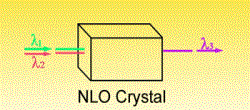Sum Frequency Generation

Frequency Doubling
Frequency Doubling or Second Harmonic Generation (SHG) is a special case of sum frequency generation if the two input wavelengths are the same: 2w1 = w2 (or l1 = 2l2 in wavelength). The simplest scheme for frequency doubling is extracavity doubling. The laser passes through the nonlinear crystal only once as shown. However, if the power density of laser is low, focused beam, intracavity doubling and external resonant cavity are normally used to increase the power density on the crystals, for example, for doubling of cw Nd:YAG laser and Argon Ion lasers. If you meet any problems in scheme selection, please contact for a solution.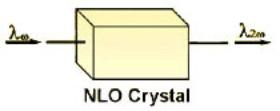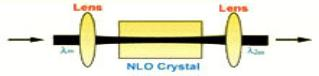Extracavity SHG                                                                 SHG With Focused Beam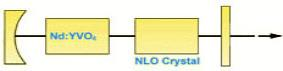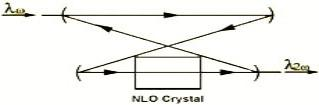Intracavity SHG                                                             External Resonant cavity SHG

Frequency Tripling
Frequency Tripling or Third Harmonic Generation (THG) is an other example of Sum Frequency Generation where, for THG of Nd:YAG laser, l1=1064nm, l2=532nm and generated wavelength l3=355nm. By sum frequency of fundamental wavelength and THG of a Ti:Sapphire laser in BBO crystal, it can generate wavelength as short as 193nm.

Differential-Frequency Generation (DFG):
ω1 - ω2 = ω3 (or 1/λ1 - 1/λ2 = 1/λ3 in wavelength) It mixes one high energy photon and one low energy photon into another low energy photon. For example:
532 nm - 810 nm -> 1550 nm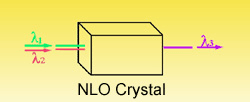Differential Frequency Generation

Optical Parametric Generation (OPG):
ωp = ωs + ωi (or 1/λp = 1/λs + 1/λi in wavelength) It splits one high-energy photon into two low-energy photons.
For example:
355 nm -> 532 nm + 1064 nm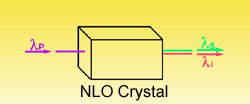Optical Parametric Generation (OPG) is an inverse process of Sum Frequency Generation. It splits one high-frequency photon (pumping wavelength, lp) into two low-frequency photons (signal, ls, and idler wavelength, li). If two mirrors are added to form a cavity as shown in following Figure, an Optical Parametric Oscillator (OPO) is established. For a fixed pump wavelength, tilting a crystal can generate an infinite number of signal and idler wavelengths. Therefore, OPO is an excellent source for generating wide tunable range coherent radiation. BBO, KTP, LBO and LiNbO3 are good crystals for OPO and Optical Parametric Amplifier (OPA) applications.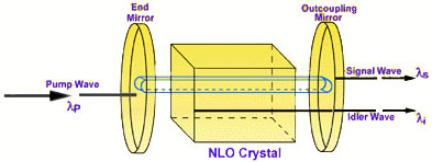Phase-Matching

In order to obtain high conversion efficiency, the phase vectors of input beams and generated beams have to be matched:
Δk = k3 - k2 - k1 = 2π33 - 2πn2/λ2 - 2πn1/λ1 = 0 (for sum frequency generation)
Where: k is phase mismatching, ki is phase vector at λi and ni is refractive index at λi.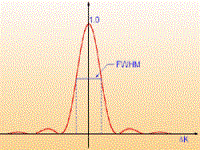Conversion Efficiency Vs Δk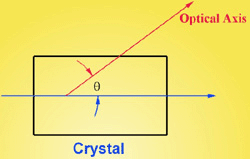The phase-matching can be obtained by angle tilting, temperature tuning or other methods. The angle tilting is mostly used to obtain phasematching as shown in the left figure. If the angle between optical axis and beam ropagation (θ) isn't equal to 90o or 0o, we call it critical phase-matching (CPM).Otherwise, 90o non-critical phasematching (NCPM) is for θ = 90o and 0o NCPM is for θ = 0o.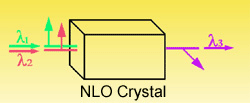Type I Phase Matching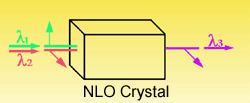Type II Phase Matching

Parameters For NLO Crystal Selection

 NLO Process Phase-Matching Type and Angle, deff Power or Energy, Repetition Rate Damage Threshold Divergence Acceptance Angle Bandwidth Spectral Spectral Acceptance Beam Size Crystal Size, Walk-Off Angle Pulse Width Group Velocity Mismatching Environment Moisture,Temperature Acceptance

Crystal Acceptance
If a laser light propagates in the direction with angle △θto phase matching direction, the conversion efficiency will reduce dramatically (see the right Figure). We define the acceptance angle (△θ) as full angle at half maximum (FAHM), where θ = 0 is phase-matching direction. For example, the acceptance angle of BBO for type I frequency doubling of Nd:YAG at 1064 nm is about 1 mrad-cm. Therefore, if a Nd:YAG laser has beam divergence of 3 mrad for frequency-doubling, over half of the input power is useless. In this case, LBO may be better because of its larger acceptance angle, about 8 mrad-cm. For NCPM, the acceptance angle is normally much bigger than that for CPM, for example, 52 mrad-cm1/2 for type I NCPM LBO.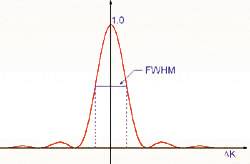In addition, you have to consider the spectral acceptance (△λ) of crystal and the spectral bandwidth of your laser; crystal temperature acceptance (△T) and the temperature change of environment
Walk-Off
Due to the birefringence of NLO crystals, the extraordinary wave (ne) will experience Poynting vector walk-off. If the beam size of input laser is small, the generated beam and input beam will be separated at a walk-off angle (ρ) in the crystal and it will cause low conversion efficiency. Therefore, for focused beam or intracavity doubling, the walk-off is a main limitation to high conversion efficiency.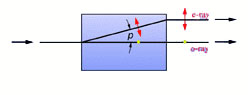Group Velocity Mismatching
For frequency conversion of ultrafast lasers such as Ti:Sapphire and Dye lasers with femtosecond (fs) pulse width, the main concern is fs pulse broadening induced by group velocity mismatching (GVM)) or group velocity dispersion of NLO crystal. In order to keep efficiency frequency conversion without significant pulse broadening, the suggested thickness (LGVM) of crystals is less than Pulse Width divides GVM. For frequency doubling a Ti:Sapphire laser at 800 nm, for example, the inverse group velocities (1/VG) of BBO are respectively 1/VG = 56.09 ps/cm at 800 nm and 1/VG = 58.01 ps/cm at 400 nm and GVM = 1.92 ps/cm. That means an 1 mm long BBO crystal will make 192 fs separation between the pulses at two wavelengths. Therefore, for an 100 fs Ti:Sapphire laser, we normally recommend a 0.5 mm long BBO crystal (with 96 fs separation) in order to obtain high efficiency without dramatic pulse broadening. SUWTECH is able to manufacture as thin as 0.02mm BBO crystals for as short as 5fs laser application.